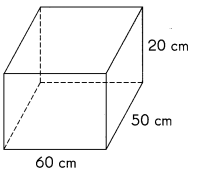# Math in Focus Grade 5 Chapter 15 Practice 6 Answer Key Volume of a Rectangular Prism and Liquid

This handy Math in Focus Grade 5 Workbook Answer Key Chapter 15 Practice 6 Volume of a Rectangular Prism and Liquid provides detailed solutions for the textbook questions.

## Math in Focus Grade 5 Chapter 15 Practice 6 Answer Key Volume of a Rectangular Prism and Liquid

Write each measure in milliliters.

Question 1.
690 cm3 = ____________
690 cm3 = 690 milliliters,

Explanation:
Given 690 cm3 to measure in milliliters,
as 1 cubic centimeter is equal to 1 milliliters
so 690 cm3 = 690 X 1 milliliters = 690 milliliters.

Question 2.
207 cm3 = ____________
207 cm3 = 207 milliliters,

Explanation:
Given 207 cm3 to measure in milliliters,
as 1 cubic centimeter is equal to 1 milliliters
so 207 cm3 = 207 X 1 milliliters = 207 milliliters.

Question 3.
2,000 cm3 = ____________
2,000 cm3 = 2,000 milliliters,

Explanation:
Given 2,000 cm3 to measure in milliliters,
as 1 cubic centimeter is equal to 1 milliliters
so 2,000 cm3 = 2,000 X 1 milliliters = 2,000 milliliters.

Question 4.
4,600 cm3 = ____________
4,600 cm3 = 4,600 milliliters,

Explanation:
Given 4,600 cm3 to measure in milliliters,
as 1 cubic centimeter is equal to 1 milliliter
so 4,600 cm3 = 4,600 X 1 milliliters = 4,600 milliliters.

Write each measure in cubic centimeters.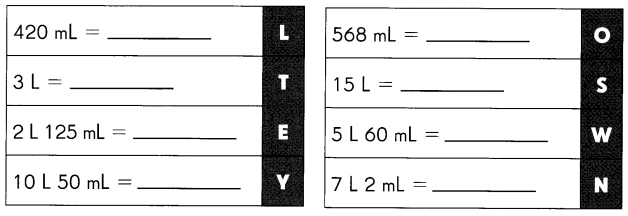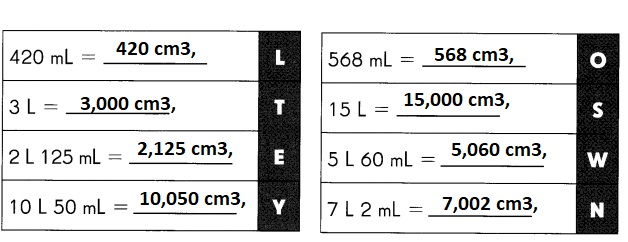Question 5.
420 mL = ________cm3,
420 ml = 420 cm3,

Explanation:
Given 420 ml to measure in cubic centimeters,
as 1 milliliters is equal to 1 cubic centimeters,
so 420 ml = 420 X 1 cubic centimeters = 420 cm3 .

Question 6.
568 mL = ____________cm3,
568 ml = 568 cm3,

Explanation:
Given 568 mL to measure in cubic centimeters,
as 1 milliliters is equal to 1 cubic centimeters,
so 568 ml = 568 X 1 cubic centimeters = 568 cm3 .

Question 7.
3 L = ____________cm3,
3 L = 3,000 X 1 ml = 3,000 cm3,

Explanation:
Given 3 L = 3,000 ml to measure in cubic centimeters,
as 1 milliliters is equal to 1 cubic centimeters,
so 3,000 ml = 3,000 X 1 cubic centimeters = 3,000 cm3 .

Question 8.
15 L = ___cm3,
15 L = 15,000 X 1 ml = 15,000 cm3,

Explanation:
Given 15 L = 15,000 ml to measure in cubic centimeters,
as 1 milliliters is equal to 1 cubic centimeters,
so 15,000 ml = 15,000 X 1 cubic centimeters = 15,000 cm3 .

Question 9.
2 L 125 mL = ___________cm3,
2 L 125 mL = 2,125 X 1 cubic centimeters = 2,125 cm3,

Explanation:
Given 2 L 125 mL = 2 ,125 mL to measure in cubic centimeters,
as 1 milliliters is equal to 1 cubic centimeters,
so 2L 125 mL = 2,125 X 1 cubic centimeters = 2,125 cm3 .

Question 10.
5 L 60 mL = ____________cm3,
5 L 60 mL = 5,060 X 1 cm = 5,060 cm3,

Explanation:
Given 5 L 60 mL = 5,060 mL to measure in cubic centimeters,
as 1 milliliters is equal to 1 cubic centimeters,
so 5 L 60 mL = 5,060 X 1 cubic centimeters = 5,060 cm3.

Question 11.
10 L 50mL = _______cm3,

Explanation:
Given 10 L 50 mL = 10,050 mL to measure in cubic centimeters,
as 1 milliliters is equal to 1 cubic centimeters,
so 10 L 50 mL = 10,050 X 1 cubic centimeters = 10,050 cm3 .

Question 12.
7 L 2mL = ____________cm3,
7 L 2 mL = 7,002 cm3,

Explanation:
Given 7 L 2 mL = 7 ,002 mL to measure in cubic centimeters,
as 1 milliliters is equal to 1 cubic centimeters,
so 7 L 2 mL = 7,002 X  1 cubic centimeters = 7,002 cm3.

Do you know which national park is the oldest in the United States?
Match the letters to the answers to find out.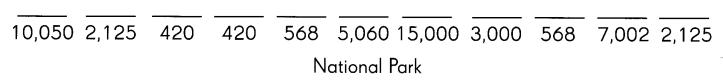YELLOW STONE  is the oldest national park in the United States,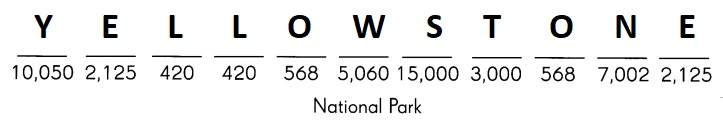Explanation:
Yes, After matching the letters the answer is YELLOW STONE,
the oldest national park in the United States.

Write each measure in liters and milliliters.

Question 13.
720 cm3 = ____________milliliters3,
720 cm3 = 720 milliliters3,

Explanation:
Given 720 cm3 to measure in cubic centimeters,
as 1 cubic centimeter is equal to 1 milliliters
so 720 cm3 = 720 X 1 cubic centimeters = 720 milliliters.

Question 14.
7,000 cm3 = _________,
7,000 cm3 = 7 L ,

Explanation:
Given 7,000 cm3 to measure in cubic centimeters,
as 1 cubic centimeter is equal to 1 milliliters and
1 liter is equal to 1000 milliliters
so 7,000 cm3 = 7,000 X 1 milliliters = 7 X 1,000 milliliters =  1 L.

Question 15.
2,050 cm3 = ___________
2,050 cm3 = 2 L 50 mL ,

Explanation:
Given 2,050 cm3 to measure in cubic centimeters,
as 1 cubic centimeter is equal to 1 milliliters and
1 liter is equal to 1000 milliliters
so 2,050 cm3 = 2,050 X 1 milliliters = 2  X 1,050 milliliters =  2 L, 50 mL.

Question 16.
1,470 cm3 = ____________
1,470 cm3 = 1 L 470 mL ,

Explanation:
Given 1,470 cm3 to measure in cubic centimeters,
as 1 cubic centimeter is equal to 1 milliliters and
1 liter is equal to 1000 milliliters
so 1,470 cm3 = 1,470 X 1 milliliters = 1  X 1,470 milliliters =  1 L, 470 mL.

Question 17.
9,801 cm3 = ____________
9,801 cm3 = 9 L 801 mL ,

Explanation:
Given 9,801 cm3 to measure in cubic centimeters,
as 1 cubic centimeter is equal to 1 milliliters and
1 liter is equal to 1000 milliliters
so 9,801 cm3 = 9,801 X 1 milliliters = 9 L X 801 milliliters =  9 L, 801 mL.

Question 18.
4,003 cm3 = ____________
4,003 cm3 = 4 L 3 mL ,

Explanation:
Given 4,003 cm3 to measure in cubic centimeters,
as 1 cubic centimeter is equal to 1 milliliters and
1 liter is equal to 1000 milliliters
so 4,003 cm3 = 4,003 X 1 milliliters = 4 L X 3 milliliters =  4 L, 3 mL.

Question 19.
10,600 cm3 = ____________
10,600 cm3 = 10,600 mL ,

Explanation:
Given 10,600 cm3 to measure in cubic centimeters,
as 1 cubic centimeter is equal to 1 milliliters and
1 liter is equal to 1000 milliliters
so 10,600 cm3 = 10,600 X 1 milliliters = 10 L X 600 milliliters =  10 L, 600 mL.

Question 20.
1,075 cm3 = _____1 L 075 mL_______
1,075 cm3 = 1 L 075 mL ,

Explanation:
Given 1,075 cm3 to measure in cubic centimeters,
as 1 cubic centimeter is equal to 1 milliliters and
1 liter is equal to 1000 milliliters
so 1,075 cm3 = 1 L X  75 milliliters = 1 L X 75 milliliters =  1 L, 75 mL.

Find the volume of water in each rectangular tank in milliliters.
(Hint: 1 cm3 = 1 mL)

Question 21.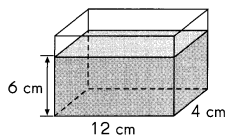Volume = ____288 mL_______
Volume = 288 mL,

Explanation:
Given rectangular tank has volume 12 cm X 4 cm X 6 cm = 288 cm3
as 1 cubic centimeter is equal to 1 milliliters,
so, 288 cm3 = 288 mL.

Question 22.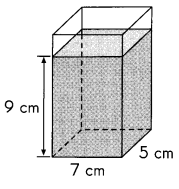Volume = ___________
Volume = 315 mL,

Explanation:
Given rectangular tank has volume as 7 cm X 5 cm X 9 cm = 315 cm3
as 1 cubic centimeter is equal to 1 milliliters,
so 315 cm3 = 315 mL.

Find the volume of water in each rectangular tank in liters and milliliters.
(Hint: 1,000 cm3 = 1 L)

Question 23.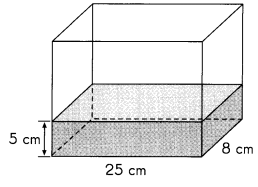Volume = ___________
Volume = 1 L,

Explanation:
Given rectangular tank has volume as 25 cm X 8 cm X 5 cm = 1000 cm3 ,
1,000 cm3 to measure in cubic centimeters,
as 1 cubic centimeter is equal to 1 milliliters and
1 liter is equal to 1000 milliliters
so 1,005 cm3 = 1,000 milliliters = 1 L.

Question 24.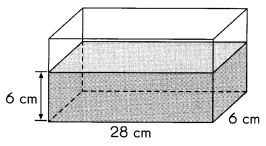Volume = ___________
Volume = 1 L 8 mL,

Explanation:
Given rectangular tank has volume as 28 cm X 6 cm X 6 cm = 1,008 cm3 ,
1,008 cm3 to measure in cubic centimeters,
as 1 cubic centimeter is equal to 1 milliliters and
1 liter is equal to 1000 milliliters
so 1,008 cm3 = 1,008 milliliters = 1 L 8 mL.

Question 25.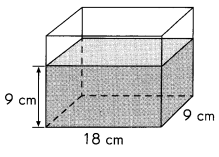Volume = ____ _______
Volume = 1 L 458 mL,

Explanation:
Given rectangular tank has volume as 18 cm X 9 cm X 9 cm = 1,458 cm3 ,
1,458 cm3 to measure in cubic centimeters,
as 1 cubic centimeter is equal to 1 milliliters and
1 liter is equal to 1000 milliliters
so 1,458 cm3 = 1,458 milliliters = 1 L 458 mL.

Question 26.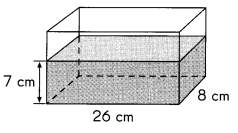Volume = ___________
Volume = 1 L 456 mL,

Explanation:
Given rectangular tank has volume as 26 cm X 8 cm X 7 cm = 1,456 cm3 ,
1,456 cm3 to measure in cubic centimeters,
as 1 cubic centimeter is equal to 1 milliliters and
1 liter is equal to 1000 milliliters
so 1,456 cm3 = 1,456 milliliters = 1 L 456 mL.

Question 27.
How much water is in this tank when it is $$\frac{1}{3}$$ full?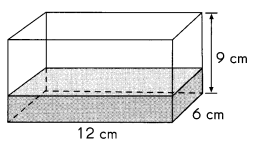The tank is filled with volume 218 cm3,

Explanation:
Given rectangular tank has volume with dimensions 12 cm X 6 cm X 9 cm = 648 cm3,
water in the tank is $$\frac{1}{3}$$,
So $$\frac{1}{3}$$ X 648 cm3 = 218 cm3.

Question 28.
This rectangular tank is filled with water to a height of 4 centimeters.
How much more water is needed to fill the tank completely?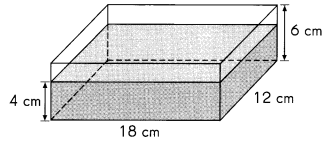Water needed to fill the tank completely is 432 cm3,

Explanation:
Given rectangular tank has volume with dimensions 18 cm X 12 cm X 6 cm = 1,296 cm3 and
tank is filled with water to a height of 4 centimeters,
so volume of tank with water filled is 18 cm X 12 cm X 4 cm = 864 cm3,
Therefore more water is needed to fill the tank completely is 1,296 cm3– 864 cm= 432 cm3.

Question 29.
A cubical tank with an edge length of 20 centimeters is filled with 3.75 liters of water.
How much more water is needed to fill the tank completely? Give your answer in liters.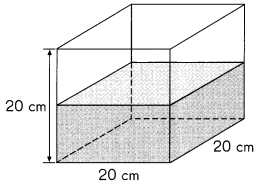More water needed to fill the tank completely is 4.25 liters,

Explanation:
Given rectangular tank has volume with dimensions 20 cm X 20 cm X 20 cm = 8,000 cm3,
So the volume of the tank in liters is 8 X 1000 mL= 8 L,
and tank cubical tank with an edge length of 20 centimeters is filled with 3.75 liters of water.
Therefore more water is needed to fill the tank completely is 8 L – 3.75 L = 4.25 liters.

Question 30.
The rectangular tank shown is $$\frac{1}{4}$$-filled with water.
Then another 1 liter 400 milliliters of water is added.
Find the volume of water in the tank in the end. Give your answer in liters and milliliters.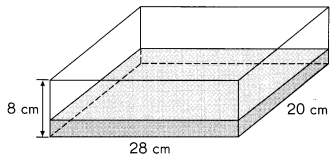The volume of water in the tank in the end is 2L 520 mL,

Explanation:
Given rectangular tank has volume with dimensions 28 cm X 20 cm X 8 cm = 4,480 cm3,
and the rectangular tank shown is $$\frac{1}{4}$$-filled with water.
So volume of tank in liters is $$\frac{1}{4}$$ X 4,480 cm3 = 1,120 cm3,
1 L 120 mL to this another 1 liter 400 milliliters of water is added and tank has
1 L 120 mL + 1L 400 mL =  2L 520  mL.

Question 31.
This container is half-filled with oil. What is the volume of oil in the container?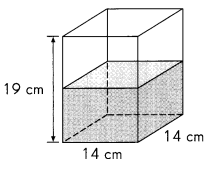The container is filled with volume 1 L 862 mL,

Explanation:
Given rectangular tank has volume with dimensions 14 cm X 14 cm X 19 cm = 3,724 cm3,
Container is half-filled with oil so volume of oil in the container is
$$\frac{1}{2}$$ X 3,724 cm3 = 1,862 cm3 = 1 L 862 mL.

Question 32.
A cubical tank whose edges each measure 1 2 centimeters is half-filled with water.
The water is poured into an empty rectangular tank measuring
10 centimeters by 8 centimeters by 7 centimeters until it is full.
How much water is left in the cubical tank? Give your answer in milliliters.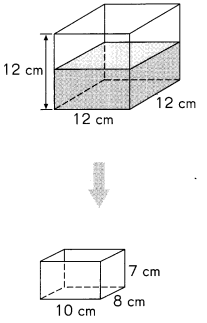Water left in the cubical tank is  1,168 cm3,

Explanation:
Given a cubical tank whose edges each measure 1 2 centimeters is half-filled with water.
So volume with dimensions 12 cm X 12 cm X 12 cm = 1,728 cm3 and
the water is poured into an empty rectangular tank measuring
10 centimeters by 8 centimeters by 7 centimeters until it is full.
So water poured is 10 cm X 8 cm X 7 cm = 560 cm3 ,
therefore, water left in the cubical tank is 1,728 cm3 – 560 cm3 = 1,168 cm3.

Question 33.
The rectangular swimming pool shown contains 600 cubic meters of water.
How much more water has to be added so that the water level is 1 meter from the top?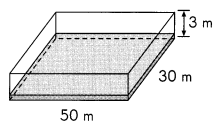Volume left in swimming pool is 4,900 cubic meters,

Explanation:
Given swimming pool contains volume of 50 m X 30 m X 3 m = 4,500 cubic meters
and the rectangular swimming pool shown contains 600 cubic meters of water.
and that the water level is 1 meter from the top is
4,500 cubic meters + 1 L = 5,500 cubic meters,
therefore, volume left in swimming pool is
5,500 cubic meters – 600 cubic meters = 4,900 cubic meters.

Question 34.
The rectangular tank shown is filled completely with water.
How much water must be taken out so the height of the water
level in the tank is 10 centimeters? Give your answer in milliliters.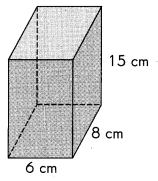Water to be taken out, so the height of the water
level in the tank is 10 centimeters is 240 cubic meters,

Explanation:
Given the rectangular tank shown is filled completely with water,
so, volume of 6 cm X 8 cm X 15 cm = 720 cubic meters,
The height of the water level in the tank is 10 centimeters,
so, volume of 6 cm X 8 cm X 10 cm = 480 cubic meters,
Water to be taken out is 720 cubic meters – 480 cubic meters = 240 cubic meters.

Question 35.
The large rectangular tank shown is $$\frac{4}{5}$$-filled with water.
The water is then poured into the smaller rectangular container until it is full.
How much water is left in the tank? Give your answer in liters and milliliters.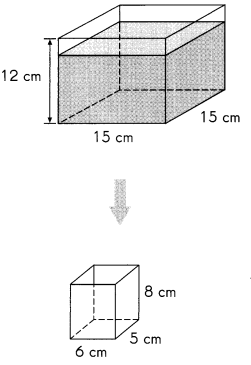Water left in the tank is 1,920 cm3,

Explanation:
Given large rectangular tank has volume with dimensions 15 cm X 15 cm X 12 cm = 2,700 cm3,
The large rectangular tank shown is $$\frac{4}{5}$$ filled with water
therefore tank has $$\frac{4}{5}$$ X 2,700 cm3 =
$$\frac{4 X 2,700}{5}$$ cm3 = 2,160 cm3.
The water is then poured into the smaller rectangular container until it is full.
Volume of smaller rectangular container with dimensions 6 cm X 5 cm X 8 cm = 2,40 cm3,
Water left in the tank is 2,160 cm3– 2,40 cm3= 1,920 cm3.

Question 36.
Water flows into this tank at 8 liters per minute. How long will it take to fill the tank?